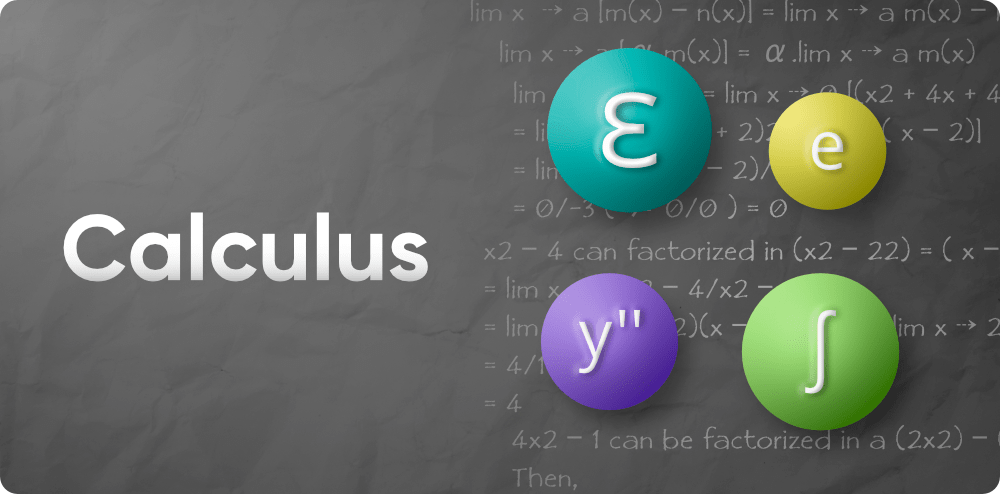# Calculus

• Difficulty Level : Easy
• Last Updated : 30 May, 2022

Calculus is a subset of mathematics concerned with the study of continuous transition. Calculus is also known as infinitesimal calculus or “infinite calculus.” The analysis of continuous change of functions is known as classical calculus. Derivatives and integrals are the two most important ideas of calculus. The integral is the measure of the region under the curve, while the derivative is the measure of the rate of change of a function. The integral accumulates the discrete values of a function over a number of values, while the derivative describes the function at a given point.Calculus, a branch of mathematics founded by Newton and Leibniz, study the pace of transition. Calculus Math is commonly used in mathematical simulations to find the best solutions. It aids us in understanding the changes between values that are linked by a purpose. Calculus Math is mostly concerned with certain critical topics such as separation, convergence, limits, functions, and so on.

Calculus Mathematics is generally divided into two types: Differential Calculus and Integral Calculus. Both differential and integral calculus consider the effect of a small shift in the independent variable on the equation as it approaches zero. Both discrete and integral calculus serves as a basis for the higher branch of mathematics known as Analysis.

### Differential Calculus

Differential Calculus deals with the issues of determining the rate of change of a parameter with respect to other variables. Derivatives are used to find the maxima and minima values of a function in order to find the best solution. The analysis of the boundary of a quotient leads to differential calculus. It is concerned with variables such as x and y, functions f(x), and the resulting variations in x and y. Differentials are represented by the symbols dy and dx. Differentiation refers to the method of determining derivatives. A function’s derivative is defined by dy/dx or f’ (x). It denotes that the equation is the derivative of y with respect to x. Let us go through some main topics discussed in simple differential calculus in the following articles:

### Integral Calculus

The analysis of integrals and their properties is known as integral calculus. It is primarily useful for the following two functions: To compute f from f’ (i.e. from its derivative). If a function f is differentiable in the range under consideration, then f’ is specified in that range. To determine the region under a curve. Differentiation is the inverse of integration. As separation can be defined as the division of a part into several small parts, integration can be defined as the selection of small parts to form a whole. It is commonly used to calculate area.

A definite integral has a specified boundary beyond which the equation must be computed. The lower and upper limits of a function’s independent variable are defined, and its integration is represented using definite integrals. An infinite integral lacks a fixed boundary, i.e. there is no upper and lower limit. As a result, the integration value is always followed by a constant value. Following are the articles that discuss the integral calculus deeply:

My Personal Notes arrow_drop_up
Recommended Articles
Page :